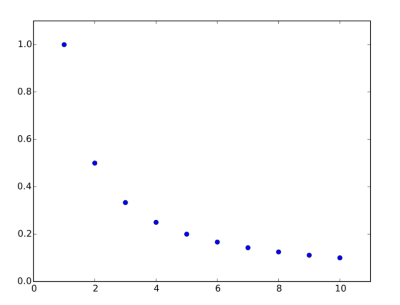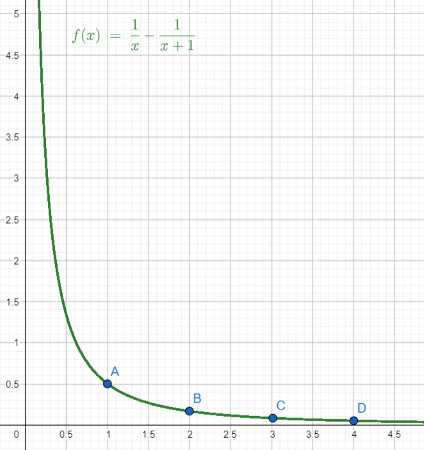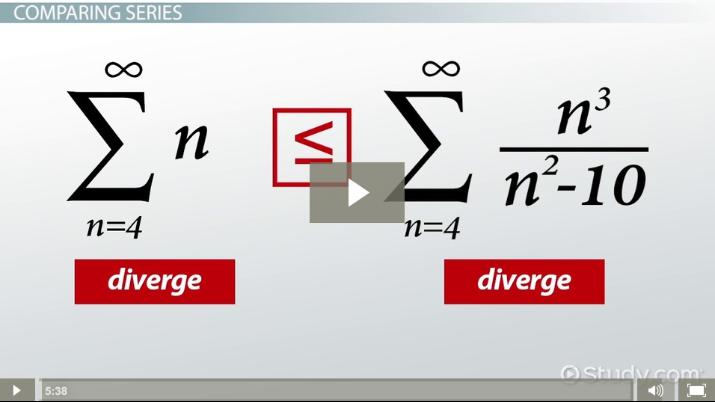# How to Determine if a Series is Convergent or Divergent

Janelle Dockter, Yuanxin (Amy) Yang Alcocer
• Author
Janelle Dockter

Janelle has taught mathematics at a middle school, high school, and collegiate level for over 5 years. She also has experience preparing students for standardized tests, writing lessons, tutoring, and grading. Janelle has a bachelor’s degree in both mathematics and computer science from University of Washington. She is enrolled in a mathematics Ph.D. program at Washington State University and is employed as a research assistant.

• Instructor
Yuanxin (Amy) Yang Alcocer

Amy has a master's degree in secondary education and has been teaching math for over 9 years. Amy has worked with students at all levels from those with special needs to those that are gifted.

Learn the convergence and divergence tests for an infinite series. See how to use comparison tests to determine if a series is convergent or divergent with examples. Updated: 02/25/2022

Show

## Infinite Series

An infinite series is the sum of an infinite sequence of numbers. Recall that a sequence is a list of numbers, often appearing as {eq}\{ a_n \} = a_1,a_2,a_3,...,a_n {/eq}. A series a sum of a sequence of terms, often appearing as {eq}\sum a_n = a_1+ a_2+ a_3+...+a_n {/eq}.

Since it can be difficult to model behavior of an infinite sum of numbers, it is helpful to consider partial sums. Partial sums are finite series, denoted {eq}S_n = a_1+a_2+a_3+...+a_n = \sum_{k=1}^n a_k {/eq}. The expression {eq}S_n {/eq} is called the n-th partial sum.

An infinite series can be found by taking the limit of the sequence of partial sums. Then, the infinite series is equal to {eq}\lim_{n \rightarrow \infty} S_n = \lim_{n \rightarrow \infty} \sum_{k=1}^n a_k {/eq}. The last part of the equation, {eq}\lim_{n \rightarrow \infty} \sum_{k=1}^n a_k {/eq}, is called the sigma notation for an infinite series. Sometimes notation is simplified, and infinite series are written as {eq}\sum_{k=1}^\infty a_k {/eq}.

### Infinite Series Examples

Some examples of infinite series are as follows.

#### Infinite Series Example 1

Let {eq}S_n = \frac{2^n -1}{2^n} {/eq}. Find the infinite series {eq}\lim_{n \rightarrow \infty} S_n {/eq}

Process

Notice that {eq}S_n = \frac{2^n - 1}{2^n} = 1 - \frac{1}{2^n} {/eq}. Next, calculate some of the partial sums, looking for the behavior of {eq}S_n {/eq} as n increases without bound.

{eq}S_1 = 1 - \frac{1}{2} = \frac{1}{2} \\ S_2 = 1 - \frac{1}{4} = \frac{3}{4}\\ S_3 = 1 - \frac{1}{8} = \frac{7}{8}\\ S_4 = 1 - \frac{1}{16} = \frac{15}{16}\\ {/eq}

Notice that as n increases, {eq}S_n {/eq} tends toward 1. Then, the limit of the sequence of partial sums is 1.

Solution

{eq}\lim_{n \rightarrow \infty} S_n = 1 {/eq}

#### Infinite Series Example 2

Let {eq}S_n = n {/eq}. Find the infinite series {eq}\lim_{n \rightarrow \infty} S_n {/eq}

Process

Notice that {eq}S_1 = 1, S_2 = 2, S_3 = 3 {/eq}.

As n increases, {eq}S_n {/eq} also increases without bounds. Then, the sequence of partial sums tends toward infinity.

Solution

{eq}\lim_{n \rightarrow \infty} \sum_{k=1}^n k = \infty {/eq}

### Convergent and Divergent Series

An infinite series will either converge to a real number, diverge to positive or negative infinity, or oscillate. The series' behavior can be found by taking the limit of the sequence of partial sums. If the limit of the sequence of partial sums is equal to a real number, the series is called a convergent series and is denoted {eq}\sum_{n=1}^\infty a_n = L {/eq}, where L is the real-valued sum. If the sequence of partial sums does not converge, the series is called a divergent series. Divergent series include infinite series that diverge to positive infinity, diverge to negative infinity, and oscillate.

#### Geometric Series

Geometric series are convergent series that take the form {eq}\sum_{k=1}^\infty ar^{k-1} = a+ar+ar^2+a^3+... {/eq}, where {eq}a \neq 0 {/eq} and {eq}r \neq 1 {/eq}. Infinite series that can be expressed as geometric series converge to {eq}\frac{a}{1-r} {/eq}.

#### P-Series

P-series are infinite series in the form {eq}\sum_{n=1}^\infty \frac{1}{n^p} {/eq}. The rule for p-series is that the infinite series diverges if {eq}p \leq 1 {/eq} and converges if {eq}p > 1 {/eq}. A series with {eq}p=1 {/eq} is called a harmonic series, which is a well-known divergent series that can be written as {eq}\sum_{n =1}^\infty \frac{1}{n} = 1 + \frac{1}{2} + \frac{1}{3} + \frac{1}{4} + ... {/eq}.Viewing the graph of the harmonic series, the sequence clearly converges to zero. The sum of the sequence, however, can be viewed in the graph as the area beneath the function. This area, while growing slowly, diverges to infinity.

#### Telescoping Sum

A telescoping sum is an infinite series with middle terms that cancel, leaving only the first and last terms in the partial sums. For example, consider the infinite series {eq}\sum_{n =1}^\infty (\frac{1}{n} - \frac{1}{n+1}) {/eq}. This series expands to:

{eq}\sum_{n =1}^\infty (\frac{1}{n} - \frac{1}{n+1}) = (1 - \frac{1}{2}) + (\frac{1}{2} - \frac{1}{3}) +(\frac{1}{3} - \frac{1}{4}) + ... \\ = \lim_{n \rightarrow \infty} [ (1 - \frac{1}{2}) + (\frac{1}{2} - \frac{1}{3}) +(\frac{1}{3} - \frac{1}{4}) + ... +(\frac{1}{n-1} - \frac{1}{n}) + (\frac{1}{n} - \frac{1}{n+1}) ] {/eq}

Notice that the middle numbers repeat negative and then positive, cancelling each set of values to zero.

{eq}\sum_{n =1}^\infty (\frac{1}{n} - \frac{1}{n+1}) = \lim_{n \rightarrow \infty} (1 - \frac{1}{n+1}) = 1 {/eq}

The last step comes from incorporating the limit at infinity, which cancels {eq}\frac{1}{n+1} {/eq} to zero. Then, this example of a telescoping sum converges to 1.#### Oscillating Series

Oscillating series are infinite series with partial sums that oscillate between two or more values. Consider the infinite series {eq}\sum_{n=1}^\infty (-1)^{n-1} = 1-1+1-1+1-1+... {/eq}. Notice that:

{eq}S_1 = 1\\ S_2 = 1-1 = 0\\ S_3 = 1-1+1 = 1\\ S_4 = 1-1+1-1 =0 {/eq}

Since the sequence of the partial sums oscillates, the limit of the sequence of partial sums does not exist. Then, the infinite series is not convergent. Since an infinite series that is not convergent is divergent, oscillating series are divergent series.

Oscillating series are less common than other forms and are not used very frequently in testing methodologies.An error occurred trying to load this video.

Try refreshing the page, or contact customer support.

Coming up next: Taylor Series: Definition, Formula & Examples

### You're on a roll. Keep up the good work!

Replay
Your next lesson will play in 10 seconds
• 0:01 Comparing Series
• 1:11 Testing for Convergence
• 2:29 Testing for Divergence
• 3:37 Example
• 4:20 Lesson Summary
Save Save

Want to watch this again later?

Timeline
Autoplay
Autoplay
Speed Speed

## Convergence and Divergence Tests

Many series do not fit the exact form of geometric series, oscillating series, p-series, or telescoping sums; one way to discern the behavior of series is to use convergence and divergence tests. The difference between the two types of tests is that divergence tests provide certain conditions for divergent series, while convergence tests provide certain conditions for convergent series. Divergence tests can never test for convergence, and convergence tests can never test for divergence.

#### Divergence Test

The divergence test is sometimes called the series divergence test or the basic divergence test. This test states that {eq}\sum_{n=1}^\infty a_n {/eq} diverges if {eq}\lim_{n\rightarrow \infty} a_n \neq 0 {/eq} or if the limit does not exist.

Note that if {eq}\lim_{n\rightarrow \infty} a_n = 0 {/eq}, the divergence test is inconclusive. In these cases, the series could be convergent, or it could still be divergent.

#### Absolute Convergence Test

The absolute convergence test states that if the sum of a sequence, {eq}\sum_{n=1}^\infty | a_n | {/eq} converges, then the sum of the sequence {eq}\sum_{n=1}^\infty a_n {/eq} also converges.

Note that if {eq}\sum_{n=1}^\infty | a_n | {/eq} does not converge, the test is inconclusive and does not give any information about {eq}\sum_{n=1}^\infty a_n {/eq}.

## Comparison Test for Convergence

If the convergence and divergence tests fail, the next step is to apply a comparison test for convergence. Comparison tests use well-known series and compare them to unknown series, allowing the evaluation of infinite series that do not fit common forms such as geometric series and p-series.

#### Comparison Test

Let {eq}\sum_{n=1}^\infty a_n {/eq} be the series in question. Let {eq}\sum_{n=1}^\infty a_n {/eq} be a series with a known solution. Let every element of each series be positive - {eq}a_n, b_n >0 {/eq}. Then, the comparison test states:

• If {eq}\sum_{n=1}^\infty b_n {/eq} converges and {eq}a_n \leq b_n {/eq} for all n, then {eq}\sum_{n=1}^\infty a_n {/eq} converges.
• If {eq}\sum_{n=1}^\infty b_n {/eq} diverges and {eq}a_n \geq b_n {/eq} for all n, then {eq}\sum_{n=1}^\infty a_n {/eq} diverges.

Note that to use the comparison test, there must be two series with all positive terms, and one series must be smaller than the other. The comparison test works by using the fact that a series will converge if it is smaller than another series that converges, and a series will diverge if it is larger than another series that diverges. Then to use this test to determine convergence of a series, it is necessary to have another larger convergent series; to use this test to determine divergence of a series, it is necessary to have another smaller divergent series.

#### Limit Comparison Test

Let {eq}\sum_{n=1}^\infty a_n {/eq} be the series in question. Let {eq}\sum_{n=1}^\infty a_n {/eq} be a series with a known solution. Let every element of each series be positive - {eq}a_n, b_n >0 {/eq}. Then, the limit comparison test states:

• If {eq}\lim_{n \rightarrow \infty} \frac{a_n}{b_n} > 0 {/eq}, then {eq}\sum_{n=1}^\infty a_n {/eq} has the same convergent or divergent behavior as {eq}\sum_{n=1}^\infty b_n {/eq}.

To unlock this lesson you must be a Study.com Member.

#### What is the difference between divergence and convergence testing?

Divergence testing only tests for divergence, while convergence testing only tests for convergence.

A divergence test will never reveal if a series is convergent, and a convergence test will never reveal if a series is divergent.

#### How do you know if a series is convergent or divergent?

First, see if the given series fits any common forms of series. The series may be a geometric series, a p-series, or a telescoping sum.

It the series does not fit a known form, begin applying divergence, convergence, and comparison tests. Begin with the divergence test. Then, check if the absolute convergence test might be applicable. If the series is similar to a known form, use the comparison test. If no other test has worked, try the limit comparison test.

#### How do you test for convergence and divergence?

Some tests will determine if a series is convergent or divergent- these tests include the limit comparison test and the comparison test. The comparison tests require the given series to be compared to a series with known convergent or divergent behavior.

Other tests will only determine convergence or only determine divergence. The divergence test provides a condition for divergent series, but the test fails if the given series does not meet this condition. The absolute convergence test provides a condition for convergent series, but the test fails if the given series does not meet this condition.

### Register to view this lesson

Are you a student or a teacher?

Back

### Resources created by teachers for teachers

Over 30,000 video lessons & teaching resources‐all in one place.Video lessonsQuizzes & WorksheetsClassroom IntegrationLesson Plans

I would definitely recommend Study.com to my colleagues. It’s like a teacher waved a magic wand and did the work for me. I feel like it’s a lifeline.

Jennifer B.
TeacherCreate an account to start this course today
Used by over 30 million students worldwide